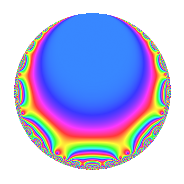# Properties

 Label 6011.2.a.dLevel 6011 Weight 2 Character orbit 6011.a Self dual Yes Analytic conductor 47.998 Analytic rank 1 Dimension 2 CM No Inner twists 1

# Related objects

## Newspace parameters

 Level: $$N$$ = $$6011$$ Weight: $$k$$ = $$2$$ Character orbit: $$[\chi]$$ = 6011.a (trivial)

## Newform invariants

 Self dual: Yes Analytic conductor: $$47.998076655$$ Analytic rank: $$1$$ Dimension: $$2$$ Coefficient field: $$\Q(\sqrt{2})$$ Coefficient ring: $$\Z[a_1, a_2]$$ Coefficient ring index: $$1$$ Fricke sign: $$1$$ Sato-Tate group: $\mathrm{SU}(2)$

## $q$-expansion

Coefficients of the $$q$$-expansion are expressed in terms of $$\beta = \sqrt{2}$$. We also show the integral $$q$$-expansion of the trace form.

 $$f(q)$$ $$=$$ $$q$$ $$+ \beta q^{2}$$ $$+ 2 q^{3}$$ $$+ 2 \beta q^{5}$$ $$+ 2 \beta q^{6}$$ $$- q^{7}$$ $$-2 \beta q^{8}$$ $$+ q^{9}$$ $$+O(q^{10})$$ $$q$$ $$+ \beta q^{2}$$ $$+ 2 q^{3}$$ $$+ 2 \beta q^{5}$$ $$+ 2 \beta q^{6}$$ $$- q^{7}$$ $$-2 \beta q^{8}$$ $$+ q^{9}$$ $$+ 4 q^{10}$$ $$-3 \beta q^{11}$$ $$+ ( -2 - \beta ) q^{13}$$ $$-\beta q^{14}$$ $$+ 4 \beta q^{15}$$ $$-4 q^{16}$$ $$-\beta q^{17}$$ $$+ \beta q^{18}$$ $$+ 2 q^{19}$$ $$-2 q^{21}$$ $$-6 q^{22}$$ $$+ ( -2 - 2 \beta ) q^{23}$$ $$-4 \beta q^{24}$$ $$+ 3 q^{25}$$ $$+ ( -2 - 2 \beta ) q^{26}$$ $$-4 q^{27}$$ $$+ 6 \beta q^{29}$$ $$+ 8 q^{30}$$ $$+ ( -4 - 3 \beta ) q^{31}$$ $$-6 \beta q^{33}$$ $$-2 q^{34}$$ $$-2 \beta q^{35}$$ $$+ ( -4 - 3 \beta ) q^{37}$$ $$+ 2 \beta q^{38}$$ $$+ ( -4 - 2 \beta ) q^{39}$$ $$-8 q^{40}$$ $$-3 q^{41}$$ $$-2 \beta q^{42}$$ $$+ 5 q^{43}$$ $$+ 2 \beta q^{45}$$ $$+ ( -4 - 2 \beta ) q^{46}$$ $$+ ( -4 + \beta ) q^{47}$$ $$-8 q^{48}$$ $$-6 q^{49}$$ $$+ 3 \beta q^{50}$$ $$-2 \beta q^{51}$$ $$-4 \beta q^{54}$$ $$-12 q^{55}$$ $$+ 2 \beta q^{56}$$ $$+ 4 q^{57}$$ $$+ 12 q^{58}$$ $$+ ( 9 - 4 \beta ) q^{59}$$ $$+ ( 2 + 2 \beta ) q^{61}$$ $$+ ( -6 - 4 \beta ) q^{62}$$ $$- q^{63}$$ $$+ 8 q^{64}$$ $$+ ( -4 - 4 \beta ) q^{65}$$ $$-12 q^{66}$$ $$+ ( 7 - 4 \beta ) q^{67}$$ $$+ ( -4 - 4 \beta ) q^{69}$$ $$-4 q^{70}$$ $$+ ( -11 - 2 \beta ) q^{71}$$ $$-2 \beta q^{72}$$ $$+ ( 8 + 2 \beta ) q^{73}$$ $$+ ( -6 - 4 \beta ) q^{74}$$ $$+ 6 q^{75}$$ $$+ 3 \beta q^{77}$$ $$+ ( -4 - 4 \beta ) q^{78}$$ $$+ ( 7 + 6 \beta ) q^{79}$$ $$-8 \beta q^{80}$$ $$-11 q^{81}$$ $$-3 \beta q^{82}$$ $$+ 9 q^{83}$$ $$-4 q^{85}$$ $$+ 5 \beta q^{86}$$ $$+ 12 \beta q^{87}$$ $$+ 12 q^{88}$$ $$+ 4 q^{90}$$ $$+ ( 2 + \beta ) q^{91}$$ $$+ ( -8 - 6 \beta ) q^{93}$$ $$+ ( 2 - 4 \beta ) q^{94}$$ $$+ 4 \beta q^{95}$$ $$+ ( -7 + 6 \beta ) q^{97}$$ $$-6 \beta q^{98}$$ $$-3 \beta q^{99}$$ $$+O(q^{100})$$ $$\operatorname{Tr}(f)(q)$$ $$=$$ $$2q$$ $$\mathstrut +\mathstrut 4q^{3}$$ $$\mathstrut -\mathstrut 2q^{7}$$ $$\mathstrut +\mathstrut 2q^{9}$$ $$\mathstrut +\mathstrut O(q^{10})$$ $$2q$$ $$\mathstrut +\mathstrut 4q^{3}$$ $$\mathstrut -\mathstrut 2q^{7}$$ $$\mathstrut +\mathstrut 2q^{9}$$ $$\mathstrut +\mathstrut 8q^{10}$$ $$\mathstrut -\mathstrut 4q^{13}$$ $$\mathstrut -\mathstrut 8q^{16}$$ $$\mathstrut +\mathstrut 4q^{19}$$ $$\mathstrut -\mathstrut 4q^{21}$$ $$\mathstrut -\mathstrut 12q^{22}$$ $$\mathstrut -\mathstrut 4q^{23}$$ $$\mathstrut +\mathstrut 6q^{25}$$ $$\mathstrut -\mathstrut 4q^{26}$$ $$\mathstrut -\mathstrut 8q^{27}$$ $$\mathstrut +\mathstrut 16q^{30}$$ $$\mathstrut -\mathstrut 8q^{31}$$ $$\mathstrut -\mathstrut 4q^{34}$$ $$\mathstrut -\mathstrut 8q^{37}$$ $$\mathstrut -\mathstrut 8q^{39}$$ $$\mathstrut -\mathstrut 16q^{40}$$ $$\mathstrut -\mathstrut 6q^{41}$$ $$\mathstrut +\mathstrut 10q^{43}$$ $$\mathstrut -\mathstrut 8q^{46}$$ $$\mathstrut -\mathstrut 8q^{47}$$ $$\mathstrut -\mathstrut 16q^{48}$$ $$\mathstrut -\mathstrut 12q^{49}$$ $$\mathstrut -\mathstrut 24q^{55}$$ $$\mathstrut +\mathstrut 8q^{57}$$ $$\mathstrut +\mathstrut 24q^{58}$$ $$\mathstrut +\mathstrut 18q^{59}$$ $$\mathstrut +\mathstrut 4q^{61}$$ $$\mathstrut -\mathstrut 12q^{62}$$ $$\mathstrut -\mathstrut 2q^{63}$$ $$\mathstrut +\mathstrut 16q^{64}$$ $$\mathstrut -\mathstrut 8q^{65}$$ $$\mathstrut -\mathstrut 24q^{66}$$ $$\mathstrut +\mathstrut 14q^{67}$$ $$\mathstrut -\mathstrut 8q^{69}$$ $$\mathstrut -\mathstrut 8q^{70}$$ $$\mathstrut -\mathstrut 22q^{71}$$ $$\mathstrut +\mathstrut 16q^{73}$$ $$\mathstrut -\mathstrut 12q^{74}$$ $$\mathstrut +\mathstrut 12q^{75}$$ $$\mathstrut -\mathstrut 8q^{78}$$ $$\mathstrut +\mathstrut 14q^{79}$$ $$\mathstrut -\mathstrut 22q^{81}$$ $$\mathstrut +\mathstrut 18q^{83}$$ $$\mathstrut -\mathstrut 8q^{85}$$ $$\mathstrut +\mathstrut 24q^{88}$$ $$\mathstrut +\mathstrut 8q^{90}$$ $$\mathstrut +\mathstrut 4q^{91}$$ $$\mathstrut -\mathstrut 16q^{93}$$ $$\mathstrut +\mathstrut 4q^{94}$$ $$\mathstrut -\mathstrut 14q^{97}$$ $$\mathstrut +\mathstrut O(q^{100})$$

## Embeddings

For each embedding $$\iota_m$$ of the coefficient field, the values $$\iota_m(a_n)$$ are shown below.

For more information on an embedded modular form you can click on its label.

Label $$\iota_m(\nu)$$ $$a_{2}$$ $$a_{3}$$ $$a_{4}$$ $$a_{5}$$ $$a_{6}$$ $$a_{7}$$ $$a_{8}$$ $$a_{9}$$ $$a_{10}$$
1.1
 −1.41421 1.41421
−1.41421 2.00000 0 −2.82843 −2.82843 −1.00000 2.82843 1.00000 4.00000
1.2 1.41421 2.00000 0 2.82843 2.82843 −1.00000 −2.82843 1.00000 4.00000
 $$n$$: e.g. 2-40 or 990-1000 Significant digits: Format: Complex embeddings Normalized embeddings Satake parameters Satake angles

## Inner twists

This newform does not admit any (nontrivial) inner twists.

## Atkin-Lehner signs

$$p$$ Sign
$$6011$$ $$1$$

## Hecke kernels

This newform can be constructed as the kernel of the linear operator $$T_{2}^{2}$$ $$\mathstrut -\mathstrut 2$$ acting on $$S_{2}^{\mathrm{new}}(\Gamma_0(6011))$$.